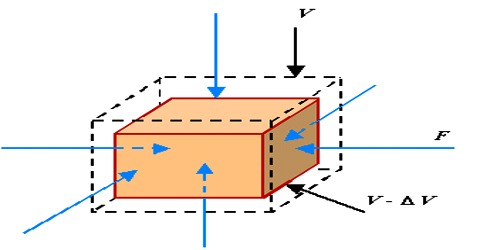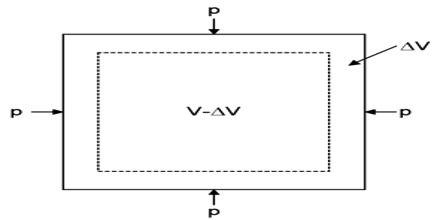# What is Volume Strain?

Volume strain: Due to the application of external force, if the volume of an elastic body is changed without changing its shape, the strain is called volume strain. It is measured by the unit change of volume.

Explanation: It is the ratio of the change in volume of a body to its original volume. If VV is the original volume of a body and V + ΔV is the volume of the body under the action of normal stress, the change in volume is ΔV.Let the initial volume of an object = V and due to application of external force the change of volume = ΔV.

So, according to definition, volume strain = change of volume / original volume = ΔV /V

So, the volumetric strain is the unit change in volume, i.e. the change in volume divided by the original volume.